SEARCH HOMEMath Central Quandaries & QueriesQuestion from Dee: In a previous question, you answered: "What percentage of $34.51 is$10.71? I translate this English statement into a mathematical statement (equation) using the following "what" is the unknown, call it x "percent" is per-one-hundred, that is over 100 "of" is multiplication "is" is the verb, which in an equation is the equal sign Thus the English statement above becomes x/100 × $34.51 =$10.71 Solving for x yields x = 31.03 Hence the gross profit is 31% of your selling price." How did you solve for 'x' ThanksHi Dee,

I had the equation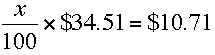Think of this as a balance with the balance point at the equal sign and the quantities on bath sides the same so that they balance. You want to manipulate both sides of the equation, always maintaining the balance and end with an equation of the form

x = some number.

I would first eliminate the fraction by multiplying both sides by 100. This will maintain the balance since you have multiplied both sides by the same amount, and yield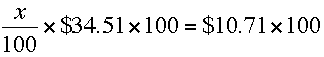You can now cancel the two 100s on the left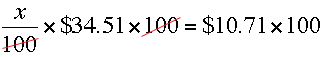to obtain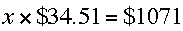Almost there. Finally divide both sides by \$34.51 which will again maintain the balance and give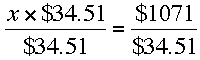Again there is cancellation on the left side, and division on the right yields

x = 31.03

I hope this helps,
PennyMath Central is supported by the University of Regina and The Pacific Institute for the Mathematical Sciences.